# Access M/J Grade 7 Mathematics (#7812020)

This document was generated on CPALMS - www.cpalms.org
You are not viewing the current course, please click the current year’s tab.

#### Course Standards

Name Description
MA.7.AR.1.1: Apply properties of operations to add and subtract linear expressions with rational coefficients.
 Clarifications:Clarification 1: Instruction includes linear expressions in the form ax±b or b±ax, where a and b are rational numbers. Clarification 2: Refer to Properties of Operations, Equality and Inequality (Appendix D).

 Examples: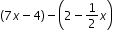is equivalent to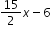.
##### Related Access Points
 Name Description MA.7.AR.1.AP.1: Add and subtract linear expressions that include like terms.

MA.7.AR.1.2: Determine whether two linear expressions are equivalent.
 Clarifications:Clarification 1: Instruction includes using properties of operations accurately and efficiently. Clarification 2: Instruction includes linear expressions in any form with rational coefficients. Clarification 3: Refer to Properties of Operations, Equality and Inequality (Appendix D).

 Examples:Are the expressions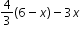and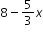equivalent?
##### Related Access Points
 Name Description MA.7.AR.1.AP.2: Use tools or manipulatives to compare two linear expressions, with no more than two operations, to determine whether they are equivalent.

MA.7.AR.2.1: Write and solve one-step inequalities in one variable within a mathematical context and represent solutions algebraically or graphically.
 Clarifications:Clarification 1: Instruction focuses on the properties of inequality. Refer to Properties of Operations, Equality and Inequality (Appendix D). Clarification 2: Instruction includes inequalities in the forms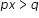;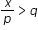; x±p>q and p±x>q, where p and q are specific rational numbers and any inequality symbol can be represented. Clarification 3: Problems include inequalities where the variable may be on either side of the inequality symbol.
##### Related Access Points
 Name Description MA.7.AR.2.AP.1: Select a one-step inequality from a list that represents a real-world situation and given a set of three or fewer values, use substitution to solve.

MA.7.AR.2.2: Write and solve two-step equations in one variable within a mathematical or real-world context, where all terms are rational numbers.
 Clarifications:Clarification 1: Instruction focuses the application of the properties of equality. Refer to Properties of Operations, Equality and Inequality (Appendix D). Clarification 2: Instruction includes equations in the forms px±q=r and p(x±q)=r, where p, q and r are specific rational numbers. Clarification 3: Problems include linear equations where the variable may be on either side of the equal sign.
##### Related Access Points
 Name Description MA.7.AR.2.AP.2a: Set up two-step equations in one variable based on real-world problems. MA.7.AR.2.AP.2b: Solve two-step equations in one variable based on real-world problems, where all terms have positive integer coefficients.

MA.7.AR.3.1: Apply previous understanding of percentages and ratios to solve multi-step real-world percent problems.
 Clarifications:Clarification 1: Instruction includes discounts, markups, simple interest, tax, tips, fees, percent increase, percent decrease and percent error.

 Examples:Example: 23% of the junior population are taking an art class this year. What is the ratio of juniors taking an art class to juniors not taking an art class? Example: The ratio of boys to girls in a class is 3:2. What percentage of the students are boys in the class?
##### Related Access Points
 Name Description MA.7.AR.3.AP.1: Solve simple percentage problems in real-world contexts.

MA.7.AR.3.2: Apply previous understanding of ratios to solve real-world problems involving proportions.
 Examples:Example: Scott is mowing lawns to earn money to buy a new gaming system and knows he needs to mow 35 lawns to earn enough money. If he can mow 4 lawns in 3 hours and 45 minutes, how long will it take him to mow 35 lawns? Assume that he can mow each lawn in the same amount of time. Example: Ashley normally runs 10-kilometer races which is about 6.2 miles. She wants to start training for a half-marathon which is 13.1 miles. How many kilometers will she run in the half-marathon? How does that compare to her normal 10K race distance?
##### Related Access Points
 Name Description MA.7.AR.3.AP.2: Solve simple ratio problems in real-world contexts.

MA.7.AR.3.3: Solve mathematical and real-world problems involving the conversion of units across different measurement systems.
 Examples:Clarification 1: Problem types are limited to length, area, weight, mass, volume and money.
##### Related Access Points
 Name Description MA.7.AR.3.AP.3: Use tools to solve real-world problems involving conversion of units in the same measurement system.

MA.7.AR.4.1: Determine whether two quantities have a proportional relationship by examining a table, graph or written description.
 Clarifications:Clarification 1: Instruction focuses on the connection to ratios and on the constant of proportionality, which is the ratio between two quantities in a proportional relationship.
##### Related Access Points
 Name Description MA.7.AR.4.AP.1: Given a table or a graph, determine whether two quantities have a proportional relationship.

MA.7.AR.4.2: Determine the constant of proportionality within a mathematical or real-world context given a table, graph or written description of a proportional relationship.
 Examples:Example: A graph has a line that goes through the origin and the point (5,2). This represents a proportional relationship and the constant of proportionality is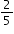. Example: Gina works as a babysitter and earns \$9 per hour. She can only work 6 hours this week. Gina wants to know how much money she will make. Gina can use the equation e=9h, where e is the amount of money earned, h is the number of hours worked and 9 is the constant of proportionality.
##### Related Access Points
 Name Description MA.7.AR.4.AP.2: Identify the constant of proportionality when given a table or graph of a proportional relationship.

MA.7.AR.4.3: Given a mathematical or real-world context, graph proportional relationships from a table, equation or a written description.
 Clarifications:Clarification 1: Instruction includes equations of proportional relationships in the form of y=px, where p is the constant of proportionality.
##### Related Access Points
 Name Description MA.7.AR.4.AP.3: Given a table or equation, graph a proportional relationship.

MA.7.AR.4.4: Given any representation of a proportional relationship, translate the representation to a written description, table or equation.
 Clarifications:Clarification 1: Given representations are limited to a written description, graph, table or equation. Clarification 2: Instruction includes equations of proportional relationships in the form of y=px, where p is the constant of proportionality.

 Examples:Example: The written description, there are 60 minutes in 1 hour, can be represented as the equation m=60h. Example: Gina works as a babysitter and earns \$9 per hour. She would like to earn \$100 to buy a new tennis racket. Gina wants to know how many hours she needs to work. She can use the equation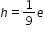, where e is the amount of money earned, h is the number of hours worked and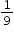is the constant of proportionality.
##### Related Access Points
 Name Description MA.7.AR.4.AP.4: Given a table representation of a proportional relationship, translate the relationship into an equation or a graph.

MA.7.AR.4.5: Solve real-world problems involving proportional relationships.
 Examples:Gordy is taking a trip from Tallahassee, FL to Portland, Maine which is about 1,407 miles. On average his SUV gets 23.1 miles per gallon on the highway and his gas tanks holds 17.5 gallons. If Gordy starts with a full tank of gas, how many times will he be required to fill the gas tank?
##### Related Access Points
 Name Description MA.7.AR.4.AP.5: Solve simple real-world problems involving proportional relationships.

MA.7.DP.1.1: Determine an appropriate measure of center or measure of variation to summarize numerical data, represented numerically or graphically, taking into consideration the context and any outliers.
 Clarifications:Clarification 1: Instruction includes recognizing whether a measure of center or measure of variation is appropriate and can be justified based on the given context or the statistical purpose. Clarification 2: Graphical representations are limited to histograms, line plots, box plots and stem-and-leaf plots. Clarification 3: The measure of center is limited to mean and median. The measure of variation is limited to range and interquartile range.
##### Related Access Points
 Name Description MA.7.DP.1.AP.1: Use context to determine the appropriate measure of center (mean or median) or range to summarize a numerical data set with 10 or fewer elements, represented numerically or graphically.

MA.7.DP.1.2: Given two numerical or graphical representations of data, use the measure(s) of center and measure(s) of variability to make comparisons, interpret results and draw conclusions about the two populations.
 Clarifications:Clarification 1: Graphical representations are limited to histograms, line plots, box plots and stem-and-leaf plots. Clarification 2: The measure of center is limited to mean and median. The measure of variation is limited to range and interquartile range.
##### Related Access Points
 Name Description MA.7.DP.1.AP.2: Given two numerical or graphical representations of data in the same form, compare the mean, median or range of each representation.

MA.7.DP.1.3: Given categorical data from a random sample, use proportional relationships to make predictions about a population.
 Examples:Example: O’Neill’s Pillow Store made 600 pillows yesterday and found that 6 were defective. If they plan to make 4,300 pillows this week, predict approximately how many pillows will be defective. Example: A school district polled 400 people to determine if it was a good idea to not have school on Friday. 30% of people responded that it was not a good idea to have school on Friday. Predict the approximate percentage of people who think it would be a good idea to have school on Friday from a population of 6,228 people.
##### Related Access Points
 Name Description MA.7.DP.1.AP.3: Given data from a random sample of the population, select from a list an appropriate prediction about the population based on the data.

MA.7.DP.1.4: Use proportional reasoning to construct, display and interpret data in circle graphs.
 Clarifications:Clarification 1: Data is limited to no more than 6 categories.
##### Related Access Points
 Name Description MA.7.DP.1.AP.4: Use proportional reasoning to interpret data in a pie chart.

MA.7.DP.1.5: Given a real-world numerical or categorical data set, choose and create an appropriate graphical representation.
 Clarifications:Clarification 1: Graphical representations are limited to histograms, bar charts, circle graphs, line plots, box plots and stem-and-leaf plots.
##### Related Access Points
 Name Description MA.7.DP.1.AP.5: Given a data set, select an appropriate graphical representation (histogram, bar chart, or line plot).

MA.7.DP.2.1: Determine the sample space for a simple experiment.
 Clarifications:Clarification 1: Simple experiments include tossing a fair coin, rolling a fair die, picking a card randomly from a deck, picking marbles randomly from a bag and spinning a fair spinner.
##### Related Access Points
 Name Description MA.7.DP.2.AP.1: Use tree diagrams, frequency tables, organized lists, and/or simulations to collect data from a simple experiment.

MA.7.DP.2.2: Given the probability of a chance event, interpret the likelihood of it occurring. Compare the probabilities of chance events.
 Clarifications:Clarification 1: Instruction includes representing probability as a fraction, percentage or decimal between 0 and 1 with probabilities close to 1 corresponding to highly likely events and probabilities close to 0 corresponding to highly unlikely events. Clarification 2: Instruction includes P(event) notation. Clarification 3: Instruction includes representing probability as a fraction, percentage or decimal.
##### Related Access Points
 Name Description MA.7.DP.2.AP.2: Given the probability of a simple chance event written as a fraction, percentage or decimal between 0 and 1, determine how likely is it that an event will occur.

MA.7.DP.2.3: Find the theoretical probability of an event related to a simple experiment.
 Clarifications:Clarification 1: Instruction includes representing probability as a fraction, percentage or decimal. Clarification 2: Simple experiments include tossing a fair coin, rolling a fair die, picking a card randomly from a deck, picking marbles randomly from a bag and spinning a fair spinner.
##### Related Access Points
 Name Description MA.7.DP.2.AP.3: Determine the theoretical probability of a simple chance event.

MA.7.DP.2.4: Use a simulation of a simple experiment to find experimental probabilities and compare them to theoretical probabilities.
 Clarifications:Clarification 1: Instruction includes representing probability as a fraction, percentage or decimal. Clarification 2: Instruction includes recognizing that experimental probabilities may differ from theoretical probabilities due to random variation. As the number of repetitions increases experimental probabilities will typically better approximate the theoretical probabilities. Clarification 3: Experiments include tossing a fair coin, rolling a fair die, picking a card randomly from a deck, picking marbles randomly from a bag and spinning a fair spinner.

 Examples:Investigate whether a coin is fair by tossing it 1,000 times and comparing the percentage of heads to the theoretical probability 0.5.
##### Related Access Points
 Name Description MA.7.DP.2.AP.4: Conduct a simple experiment to find experimental probabilities.

MA.7.GR.1.1: Apply formulas to find the areas of trapezoids, parallelograms and rhombi.
 Clarifications:Clarification 1: Instruction focuses on the connection from the areas of trapezoids, parallelograms and rhombi to the areas of rectangles or triangles. Clarification 2: Within this benchmark, the expectation is not to memorize area formulas for trapezoids, parallelograms and rhombi.
##### Related Access Points
 Name Description MA.7.GR.1.AP.1: Given the formulas, find the area of parallelograms and rhombi.

MA.7.GR.1.2: Solve mathematical or real-world problems involving the area of polygons or composite figures by decomposing them into triangles or quadrilaterals.
 Clarifications:Clarification 1: Within this benchmark, the expectation is not to find areas of figures on the coordinate plane or to find missing dimensions.
##### Related Access Points
 Name Description MA.7.GR.1.AP.2: Decompose complex shapes (polygon, trapezoid, and pentagon) into simple shapes (rectangles, squares, triangles) to measure area.

MA.7.GR.1.3: Explore the proportional relationship between circumferences and diameters of circles. Apply a formula for the circumference of a circle to solve mathematical and real-world problems.
 Clarifications:Clarification 1: Instruction includes the exploration and analysis of circular objects to examine the proportional relationship between circumference and diameter and arrive at an approximation of pi (π) as the constant of proportionality. Clarification 2: Solutions may be represented in terms of pi (π) or approximately.
##### Related Access Points
 Name Description MA.7.GR.1.AP.3: Apply a given formula for the circumference of a circle to solve mathematical problems.

MA.7.GR.1.4: Explore and apply a formula to find the area of a circle to solve mathematical and real-world problems.
 Clarifications:Clarification 1: Instruction focuses on the connection between formulas for the area of a rectangle and the area of a circle. Clarification 2: Problem types include finding areas of fractional parts of a circle. Clarification 3: Solutions may be represented in terms of pi (π) or approximately.

 Examples:If a 12-inch pizza is cut into 6 equal slices and Mikel ate 2 slices, how many square inches of pizza did he eat?
##### Related Access Points
 Name Description MA.7.GR.1.AP.4: Apply a given formula to find the area of a circle to solve mathematical problems.

MA.7.GR.1.5: Solve mathematical and real-world problems involving dimensions and areas of geometric figures, including scale drawings and scale factors.
 Clarifications:Clarification 1: Instruction focuses on seeing the scale factor as a constant of proportionality between corresponding lengths in the scale drawing and the original object. Clarification 2: Instruction includes the understanding that if the scaling factor is k, then the constant of proportionality between corresponding areas is k² . Clarification 3: Problem types include finding the scale factor given a set of dimensions as well as finding dimensions when given a scale factor.
##### Related Access Points
 Name Description MA.7.GR.1.AP.5: Use a scale factor to draw a scale drawing of a real-world two-dimensional polygon on graph paper.

MA.7.GR.2.1: Given a mathematical or real-world context, find the surface area of a right circular cylinder using the figure’s net.
 Clarifications:Clarification 1: Instruction focuses on representing a right circular cylinder with its net and on the connection between surface area of a figure and its net. Clarification 2: Within this benchmark, the expectation is to find the surface area when given a net or when given a three-dimensional figure. Clarification 3: Within this benchmark, the expectation is not to memorize the surface area formula for a right circular cylinder. Clarification 4: Solutions may be represented in terms of pi (π) or approximately.
##### Related Access Points
 Name Description MA.7.GR.2.AP.1: Match the parts of a given formula to the right circular cylinder using the figure’s net.

MA.7.GR.2.2: Solve real-world problems involving surface area of right circular cylinders.
 Clarifications:Clarification 1: Within this benchmark, the expectation is not to memorize the surface area formula for a right circular cylinder or to find radius as a missing dimension. Clarification 2: Solutions may be represented in terms of pi (π) or approximately.
##### Related Access Points
 Name Description MA.7.GR.2.AP.2: Given the formula, use tools to find the surface area of a right circular cylinder using the figure’s net.

MA.7.GR.2.3: Solve mathematical and real-world problems involving volume of right circular cylinders.
 Clarifications:Clarification 1: Within this benchmark, the expectation is not to memorize the volume formula for a right circular cylinder or to find radius as a missing dimension. Clarification 2: Solutions may be represented in terms of pi (π) or approximately.
##### Related Access Points
 Name Description MA.7.GR.2.AP.3: Given a formula, use tools to calculate the volume of right circular cylinders.

MA.7.NSO.1.1: Know and apply the Laws of Exponents to evaluate numerical expressions and generate equivalent numerical expressions, limited to whole-number exponents and rational number bases.
 Clarifications:Clarification 1: Instruction focuses on building the Laws of Exponents from specific examples. Refer to the K-12 Formulas (Appendix E) for the Laws of Exponents. Clarification 2: Problems in the form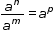must result in a whole-number value for p.
##### Related Access Points
 Name Description MA.7.NSO.1.AP.1: Use properties of whole number exponents to produce equivalent expressions.

MA.7.NSO.1.2: Rewrite rational numbers in different but equivalent forms including fractions, mixed numbers, repeating decimals and percentages to solve mathematical and real-world problems.
 Examples:Justin is solving a problem where he computes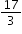and his calculator gives him the answer 5.6666666667. Justin makes the statement that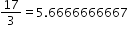; is he correct?
##### Related Access Points
 Name Description MA.7.NSO.1.AP.2: Rewrite positive rational numbers in different but equivalent forms such as fractions, mixed numbers, repeating decimals and/or percentages to solve problems.

MA.7.NSO.2.1: Solve mathematical problems using multi-step order of operations with rational numbers including grouping symbols, whole-number exponents and absolute value.
 Clarifications:Clarification 1: Multi-step expressions are limited to 6 or fewer steps.
##### Related Access Points
 Name Description MA.7.NSO.2.AP.1: Solve mathematical problems, using no more than four operations, with rational numbers including grouping symbols, whole-number exponents and absolute value.

MA.7.NSO.2.2: Add, subtract, multiply and divide rational numbers with procedural fluency.
##### Related Access Points
 Name Description MA.7.NSO.2.AP.2: Using tools or models, add, subtract, multiply and divide rational numbers.

MA.7.NSO.2.3: Solve real-world problems involving any of the four operations with rational numbers.
 Clarifications:Clarification 1: Instruction includes using one or more operations to solve problems.
##### Related Access Points
 Name Description MA.7.NSO.2.AP.3: Using tools or models, solve real-world problems involving any of the four operations with rational numbers.

MA.K12.MTR.1.1: Actively participate in effortful learning both individually and collectively.

Mathematicians who participate in effortful learning both individually and with others:

• Analyze the problem in a way that makes sense given the task.
• Build perseverance by modifying methods as needed while solving a challenging task.
• Stay engaged and maintain a positive mindset when working to solve tasks.
• Help and support each other when attempting a new method or approach.

 Clarifications:Teachers who encourage students to participate actively in effortful learning both individually and with others: Cultivate a community of growth mindset learners.  Foster perseverance in students by choosing tasks that are challenging. Develop students’ ability to analyze and problem solve. Recognize students’ effort when solving challenging problems.
MA.K12.MTR.2.1: Demonstrate understanding by representing problems in multiple ways.

Mathematicians who demonstrate understanding by representing problems in multiple ways:

• Build understanding through modeling and using manipulatives.
• Represent solutions to problems in multiple ways using objects, drawings, tables, graphs and equations.
• Progress from modeling problems with objects and drawings to using algorithms and equations.
• Express connections between concepts and representations.
• Choose a representation based on the given context or purpose.
 Clarifications:Teachers who encourage students to demonstrate understanding by representing problems in multiple ways: Help students make connections between concepts and representations.Provide opportunities for students to use manipulatives when investigating concepts.Guide students from concrete to pictorial to abstract representations as understanding progresses.Show students that various representations can have different purposes and can be useful in different situations.
MA.K12.MTR.3.1: Complete tasks with mathematical fluency.

Mathematicians who complete tasks with mathematical fluency:

• Select efficient and appropriate methods for solving problems within the given context.
• Maintain flexibility and accuracy while performing procedures and mental calculations.
• Complete tasks accurately and with confidence.
• Adapt procedures to apply them to a new context.
• Use feedback to improve efficiency when performing calculations.
 Clarifications:Teachers who encourage students to complete tasks with mathematical fluency:Provide students with the flexibility to solve problems by selecting a procedure that allows them to solve efficiently and accurately.Offer multiple opportunities for students to practice efficient and generalizable methods.Provide opportunities for students to reflect on the method they used and determine if a more efficient method could have been used.
MA.K12.MTR.4.1: Engage in discussions that reflect on the mathematical thinking of self and others.

Mathematicians who engage in discussions that reflect on the mathematical thinking of self and others:

• Communicate mathematical ideas, vocabulary and methods effectively.
• Analyze the mathematical thinking of others.
• Compare the efficiency of a method to those expressed by others.
• Recognize errors and suggest how to correctly solve the task.
• Justify results by explaining methods and processes.
• Construct possible arguments based on evidence.
 Clarifications:Teachers who encourage students to engage in discussions that reflect on the mathematical thinking of self and others:Establish a culture in which students ask questions of the teacher and their peers, and error is an opportunity for learning.Create opportunities for students to discuss their thinking with peers.Select, sequence and present student work to advance and deepen understanding of correct and increasingly efficient methods.Develop students’ ability to justify methods and compare their responses to the responses of their peers.
MA.K12.MTR.5.1: Use patterns and structure to help understand and connect mathematical concepts.

Mathematicians who use patterns and structure to help understand and connect mathematical concepts:

• Focus on relevant details within a problem.
• Create plans and procedures to logically order events, steps or ideas to solve problems.
• Decompose a complex problem into manageable parts.
• Relate previously learned concepts to new concepts.
• Look for similarities among problems.
• Connect solutions of problems to more complicated large-scale situations.
 Clarifications:Teachers who encourage students to use patterns and structure to help understand and connect mathematical concepts:Help students recognize the patterns in the world around them and connect these patterns to mathematical concepts.Support students to develop generalizations based on the similarities found among problems.Provide opportunities for students to create plans and procedures to solve problems.Develop students’ ability to construct relationships between their current understanding and more sophisticated ways of thinking.
MA.K12.MTR.6.1: Assess the reasonableness of solutions.

Mathematicians who assess the reasonableness of solutions:

• Estimate to discover possible solutions.
• Use benchmark quantities to determine if a solution makes sense.
• Check calculations when solving problems.
• Verify possible solutions by explaining the methods used.
• Evaluate results based on the given context.
 Clarifications:Teachers who encourage students to assess the reasonableness of solutions:Have students estimate or predict solutions prior to solving.Prompt students to continually ask, “Does this solution make sense? How do you know?”Reinforce that students check their work as they progress within and after a task.Strengthen students’ ability to verify solutions through justifications.
MA.K12.MTR.7.1: Apply mathematics to real-world contexts.

Mathematicians who apply mathematics to real-world contexts:

• Connect mathematical concepts to everyday experiences.
• Use models and methods to understand, represent and solve problems.
• Perform investigations to gather data or determine if a method is appropriate. • Redesign models and methods to improve accuracy or efficiency.
 Clarifications:Teachers who encourage students to apply mathematics to real-world contexts:Provide opportunities for students to create models, both concrete and abstract, and perform investigations.Challenge students to question the accuracy of their models and methods.Support students as they validate conclusions by comparing them to the given situation.Indicate how various concepts can be applied to other disciplines.
ELA.K12.EE.1.1: Cite evidence to explain and justify reasoning.
 Clarifications:K-1 Students include textual evidence in their oral communication with guidance and support from adults. The evidence can consist of details from the text without naming the text. During 1st grade, students learn how to incorporate the evidence in their writing.2-3 Students include relevant textual evidence in their written and oral communication. Students should name the text when they refer to it. In 3rd grade, students should use a combination of direct and indirect citations.4-5 Students continue with previous skills and reference comments made by speakers and peers. Students cite texts that they’ve directly quoted, paraphrased, or used for information. When writing, students will use the form of citation dictated by the instructor or the style guide referenced by the instructor. 6-8 Students continue with previous skills and use a style guide to create a proper citation.9-12 Students continue with previous skills and should be aware of existing style guides and the ways in which they differ.
 Clarifications:See Text Complexity for grade-level complexity bands and a text complexity rubric.
ELA.K12.EE.3.1: Make inferences to support comprehension.
 Clarifications:Students will make inferences before the words infer or inference are introduced. Kindergarten students will answer questions like “Why is the girl smiling?” or make predictions about what will happen based on the title page. Students will use the terms and apply them in 2nd grade and beyond.
ELA.K12.EE.4.1: Use appropriate collaborative techniques and active listening skills when engaging in discussions in a variety of situations.
 Clarifications:In kindergarten, students learn to listen to one another respectfully.In grades 1-2, students build upon these skills by justifying what they are thinking. For example: “I think ________ because _______.” The collaborative conversations are becoming academic conversations.In grades 3-12, students engage in academic conversations discussing claims and justifying their reasoning, refining and applying skills. Students build on ideas, propel the conversation, and support claims and counterclaims with evidence.
ELA.K12.EE.5.1: Use the accepted rules governing a specific format to create quality work.
 Clarifications:Students will incorporate skills learned into work products to produce quality work. For students to incorporate these skills appropriately, they must receive instruction. A 3rd grade student creating a poster board display must have instruction in how to effectively present information to do quality work.
ELA.K12.EE.6.1: Use appropriate voice and tone when speaking or writing.
 Clarifications:In kindergarten and 1st grade, students learn the difference between formal and informal language. For example, the way we talk to our friends differs from the way we speak to adults. In 2nd grade and beyond, students practice appropriate social and academic language to discuss texts.
ELD.K12.ELL.MA.1: English language learners communicate information, ideas and concepts necessary for academic success in the content area of Mathematics.
ELD.K12.ELL.SI.1: English language learners communicate for social and instructional purposes within the school setting.

## General Course Information and Notes

### VERSION DESCRIPTION

Access Courses:

Access courses are for students with the most significant cognitive disabilities. Access courses are designed to provide students access to grade-level general curriculum. Access points are alternate academic achievement standards included in access courses that target the salient content of Florida’s standards. Access points are intentionally designed to academically challenge students with the most significant cognitive disabilities.

### GENERAL NOTES

English Language Development ELD Standards Special Notes Section:

Teachers are required to provide listening, speaking, reading and writing instruction that allows English language learners (ELL) to communicate information, ideas and concepts for academic success in the content area of Language Arts. For the given level of English language proficiency and with visual, graphic, or interactive support, students will interact with grade level words, expressions, sentences and discourse to process or produce language necessary for academic success. The ELD standard should specify a relevant content area concept or topic of study chosen by curriculum developers and teachers which maximizes an ELL's need for communication and social skills. To access an ELL supporting document which delineates performance definitions and descriptors, please click on the following link: https://cpalmsmediaprod.blob.core.windows.net/uploads/docs/standards/eld/la.pdf.

### General Information

 Course Number: 7812020 Course Path: Section: Exceptional Student Education > Grade Group: Middle/Junior High > Subject: Academics - Subject Areas > Abbreviated Title: ACCESS M/J GR 7 MATH Course Attributes: Class Size Core Required Florida Standards Course Course Type: Core Academic Course Course Status: Draft - Course Pending Approval